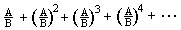Sarah, age 7, said to me unsolicited, after working on an infinite series in Ch. 1: "At school when we do math we use different methods that I am not used to. And they are hard. But if I used my own methods it would be easy." -The wisdom of young people!
_________________________________________________________

Choose Sample Problems from Chapters in Don's "Calculus By and For Young People - Worksheets" & "Changing Shapes With Matrices" books below

Check out, and click on, Don's A Map to Calculus' to see how all the student work and Don's books and videos fit into the scheme of things.

- just click on the Chapter # for sample problems:
Chapter 1: 7 Year-Olds DoChapter 3: Ian's Proof: Infinity = -1
Chapter 4: The Snowflake Curve--It's Area and Perimeter
Chapter 5: The Harmonic Series
Chapter 6: On Thin Spaghetti and Nocturnal Animals (functions and graphs)
Chapter 7: The Fibonacci Numbers, Pineapples, Sunflowers and The Golden Mean
Chapter 8: Solving Equations and Iteration
Chapter 9: The Binomial Expansion and Infinite Series
Chapter 10: Pi and Square Roots
Chapter 11: Compound Interest to e and i
Chapter 12: The Two Problems of The Calculus
Chapter 13: Area Under Curves--The Integral
Chapter 14: Slopes and The Derivative
CSWM
Changing Shapes With matrices

To see some puzzles, games and other hand's on math things Don uses